# BIOSTATISTICS

• ARITHMETIC MEAN

• The arithmetic mean is the average of a group of numbers. It is what most people think of when they hear the word "average."
• To calculate the arithmetic mean of a group of values, sum the values and then divide by the number of values

• CORRELATION

• Covariance
• Covariance is a quantitative measure of association between two variables
• For each value of x and y, the mean of the variable is subtracted from the value and then the product of the 2 values is calculated. The covariance is the average of the products.

•• If the two variables x and y are independent (have no association), then the covariance will be around 0 because about half the products will be positive and half will be negative, and when summed, they will cancel each other out
• If large values of one variable tend to occur with large values of the other variable, then the two variables will have a positive covariance
• If large values of one variable tend to occur with small values of the other variable, then the two variables will have a negative covariance

• Correlation coefficient (r)
• Covariance is expressed in units of one variable multiplied by another, and typically, this has no intuitive meaning
• In order to standardize the covariance and make it more intuitive, the correlation coefficient can be computed
• The correlation coefficient is calculated by taking the covariance and dividing it by the product of each variable's standard deviation
• The correlation coefficient is often denoted by the letter r. It is also referred to as the Pearson correlation coefficient.

•• The correlation coefficient always has a value between -1 and 1 because the covariance can never exceed the product of the standard deviations
• If two variables are perfectly positively correlated, then they will have a correlation coefficient of 1
• If two variables are perfectly negatively correlated, then they will have a correlation coefficient of -1
• If two variables have no correlation, then they will have a correlation coefficient around 0

• Coefficient of determination (r2)
• The coefficient of determination is the square of the correlation coefficient. It is often denoted as r2.
• The coefficient of determination represents the portion of the variance in the dependent variable that is explained by the independent variable
• In terms of regression analysis, it is the Regression SS/Total SS
• r2 always lies between 0 and 1. If x and y are perfectly related, meaning all the variance in y can be explained by x, then r2 will equal 1. If x and y are completely unrelated, then x will explain none of the variance of y, and r2 will be 0.

• COX PROPORTIONAL-HAZARD

• Overview
• The Cox proportional-hazard is a test used to compare survival data
• It is frequently used to analyze covariates in survival data
• The log-rank test can be used to analyze covariates by stratifying data across each covariate. If there are many covariates and strata, or if a covariate is continuous, then the log-rank test is less powerful, and the cox proportional-hazard is preferred.
• The Cox proportional-hazards model is calculated with the formula below

•• An intervention (or exposure) can be treated as an independent variable in the proportional-hazard model
• The coefficients (B) in the formula are derived using logistic regression techniques
• Logistic regression techniques involve using maximum likelihood estimations to derive regression coefficients
• The test for significance can be done on the regression coefficients (B) which follow a Z-distribution

• DATA TYPES

• Overview
• There are four basic types of data encountered in biostatistics - nominal, ordinal, interval, and ratio
• It's important to consider the different types of data, because the data type dictates which statistical tests are used to evaluate the data

• Nominal data
• Nominal data is categorical data that has no inherent or meaningful order
• Examples of nominal data include the following:
• Sex - male, female
• Race - white, black, hispanic, asian
• Medical conditions - diabetic vs nondiabetic, hypertensive vs nonhypertensive, etc.

• Ordinal data
• Ordinal data is categorical data that can be ordered, but the difference between categories has no inherent numerical value
• A common example of ordinal data is a pain scale that goes from 1 (no pain) to 10 (severe pain)
• The difference between 2 and 4 is two points, and the difference between 8 and 10 is two points. Even though the difference is the same, there is no way to prove that the two point difference has a consistent and meaningful value across the entire scale. Furthermore, people perceive pain differently, so a pain score of 5 for one patient may be a 3 for another patient. The scale has no uniformity across subjects.

• Interval data
• Interval data is data where the difference between values has meaning, but the zero point is arbitrary
• An example of interval data is temperature. The difference between 90° and 100° is 10°. The difference between 40° and 50° is 10°. This difference of 10° has numerical meaning and can be measured consistently across the entire scale. The set point of 0° however, is arbitrary. Zero degrees has a different meaning across different scales (Fahrenheit, Celsius, Kelvin). 100° is not twice as hot as 50° because 0° is arbitrary. For example, if a set point of 200° had been chosen instead of 0°, then 300° (200° + 100°) would not be twice as hot as 250° (200° + 50°). This distinction is what sets interval data apart from ratio data. Because zero is arbitrary, their ratios cannot be compared.

• Ratio data
• Ratio data is data where the difference between values has meaning, and zero has a defined value
• An example of ratio data is weight. Differences between weights have numerical meaning and can be measured consistently across the entire scale. Zero weight has a consistent meaning across different measures of weight (pounds, kilograms, etc.). Because zero is not arbitrary, weight ratios have meaning and can be compared. For example, 20 pounds is twice as much as 10 pounds, and so on.

• Cardinal data
• Interval and ratio data are sometimes collectively referred to as cardinal data

• DEGREES OF FREEDOM

• Degrees of freedom is the number of independent pieces of information on which an estimate is based
• Degrees of freedom is best explained using examples

• Example 1
• Suppose you want to estimate the standard deviation of birth weights in Dallas, Texas
• One might randomly sample 100 birth weights from various hospitals around Dallas
• The formula for calculating the standard deviation is:

•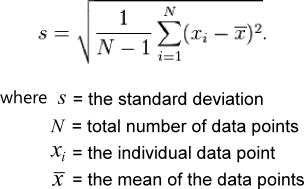• In our example, N = 100 (100 birth weights)
• In order to calculate the standard deviation, we must first calculate the mean of the birth weights ()
• The mean of the birth weights () was not measured directly, it was estimated by our calculation
• The standard deviation in our example has 99 degrees of freedom: independent pieces of information = 100; estimated pieces of information = 1; degrees of freedom = 100 - 1 = 99
• "N - 1" in the formula above represents degrees of freedom

• F DISTRIBUTION

• Distribution: ratio of the variance of two groups; distribution varies depending on the degrees of freedom in the numerator and denominator
• Number of samples: 2 or more
• Uses: to compare the variances of two samples; ANOVA testing; to compare regression models for goodness of fit
• Sample relation: Independent
• The F distribution is a continuous distribution that is used to compare the variance of two or more samples. The test statistic for an F distribution is derived from a ratio of the variances.
• The shape of the F distribution varies depending on the degrees of freedom (df) in the two samples. Because the test statistic is a ratio, the degrees of freedom (df) are expressed as numerator df and denominator df.
• As the degrees of freedom increase in either one or both samples (when denominator df ≥ 3), the critical value of the test statistic approaches 1
• The F distribution is mostly used in ANOVA testing that involves 3 or more samples. It can also be used to compare the variance of two samples and to compare regression models for goodness of fit.
• Examples of different F distributions:
•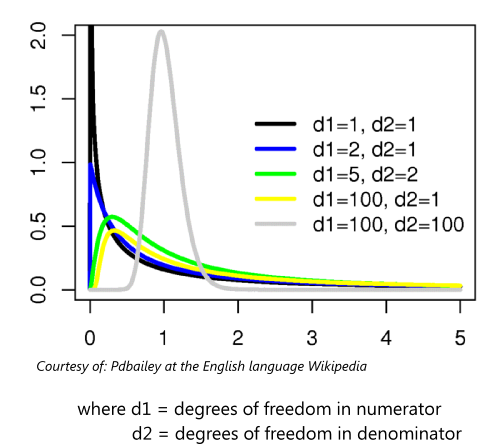• The probability density function for the F distribution is:

•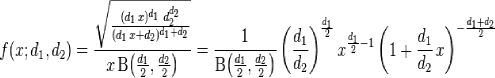• FISHER'S EXACT TEST

• Distribution: None, calculates exact probability
• Outcome: Binomial
• Number of samples: 2
• Sample relation: Independent
• Fisher's exact test is a method used to compare binomial outcomes between independent samples
• Fisher's exact test calculates the exact probability of the observed outcomes, so assumptions about the underlying distribution do not have to be valid
• If a contingency table is constructed from a set of data, and one of the cells has a value < 5, then Fisher's exact test is often used to determine the probability of the observed table. It can also be used for any set of data, regardless of the cell count.
• Fisher's exact test uses the hypergeometric distribution. The hypergeometric distribution assumes that each observation is not independent because the number of successes in the population is fixed. This means after one success is selected, the probability of the next selection being a success is lowered. For example, if a population of 16 has 9 successes, then the probability of selecting a success is 9/16. After one success is selected, the probability of selecting a success then becomes 8/15.
• Fisher's exact test is calculated in the following manner:
•• GEOMETRIC MEAN

• The geometric mean is another measure of central tendency that is useful in several scenarios
• When a group of values appear to have a skewed distribution which often means they are related to each other in a multiplicative fashion (not linear), then the geometric mean is a better measure of their average [1,2]

• The formula for calculating the geometric mean can be written in one of two ways:

•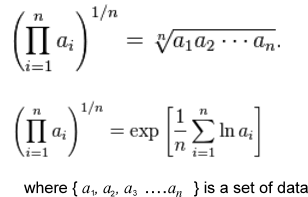• Example of using the geometric mean

•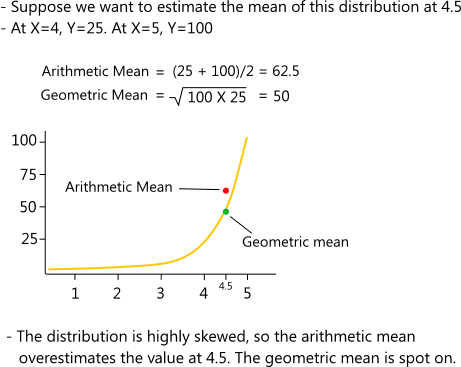• The geometric mean also facilitates the comparison of two means that are derived from values that use a different scale
• Below is an excellent example from Wikipedia of this use
• "The geometric mean can give a meaningful "average" to compare two companies which are each rated at 0 to 5 for their environmental sustainability, and are rated at 0 to 100 for their financial viability. If an arithmetic mean was used instead of a geometric mean, the financial viability is given more weight because its numeric range is larger- so a small percentage change in the financial rating (e.g. going from 80 to 90) makes a much larger difference in the arithmetic mean than a large percentage change in environmental sustainability (e.g. going from 2 to 5). The use of a geometric mean "normalizes" the ranges being averaged, so that no range dominates the weighting, and a given percentage change in any of the properties has the same effect on the geometric mean. So, a 20% change in environmental sustainability from 4 to 4.8 has the same effect on the geometric mean as a 20% change in financial viability from 60 to 72.
• The geometric mean can be understood in terms of geometry. The geometric mean of two numbers, a and b, is the length of one side of a square whose area is equal to the area of a rectangle with sides of lengths a and b."

• KAPLAN-MEIER ESTIMATOR

• Overview
• Survival curves are used to compare the probability of survival over time between two groups
• One group is typically given an intervention, and the other groups is not (control). The two groups are then followed for a period of time and the incidence of death in each group is measured. The two groups are then compared to each other.
• Survival curves are not limited to comparing mortality. They are also frequently used to compare the occurrence of an event (ex. heart attack, cancer recurrence) between two groups.

• Kaplan-Meier estimator
• One of the most common methods for creating a survival curve is the Kaplan-Meier estimator (also called the product-limit estimate)
• To construct a Kaplan-Meier survival curve, a group of subjects is followed for a period of time. The time period is then divided into intervals (ex. day, month, etc.) The probability of surviving to each consecutive interval is then calculated.
• Subjects who drop out of the study are called "censored." They are considered noninformative and assumed to have the same underlying survival curve as the uncensored subjects. In our examples, censored subjects are also assumed to have survived up to the end of the interval in which they were censored. There are calculations that make other assumptions.
• Calculating a Kaplan-Meier survival curve
• 1. Follow two groups of subjects for a period of time
• 2. Record when subjects die (or have an event) or when they drop out of the study or are lost to follow-up (censored)
• 3. Divide the time period they were followed into intervals. NOTE: Intervals do not have to be equal lengths. Typically, a new time period begins with each subsequent death (or event). If the study is very large, then the intervals may be divided into days, weeks, or months.
• 4. For each interval, calculate the probability of survival. Subjects who are censored during an interval are considered to have survived to the beginning of the interval, but are not counted in the actual interval where they were censored. NOTE: There are other methods of addressing censored subjects which doesn't follow this exactly.
• 5. Multiply the probabilities of surviving each interval

• Formula for Kaplan-Meier estimator

•• Comparing Kaplan-Meier curves
• After a kaplan-meier curve is created for each group, the curves will need to be compared to each other to see if there is a significant difference between the groups
• Two ways to compare survival curves:
• 1. Compare the curves at a selected time point
• 2. Compare the total curves

• Comparing the curves at selected time points
• Two Kaplan-Meier curves can be compared at a selected time point
• To do this, the variance of the curves is first estimated (typically with the Greenwood formula)
• A Z-test for significance is then performed
• Greenwood formula for estimating the variance of a survival curve

•• Z-test for comparing survival curves at selected time points

•• Comparing the curve at selected time points is useful in shorter studies where investigators are interested in simple outcomes like survival (or events) at one year
• It's important to make sure that the time point makes sense and that it was clearly defined before the trial was performed. Selecting time points post hoc can lead to problems, because investigators may look at survival curves and select time points where they know the curves are significantly different.

• Comparing the total curves
• In most circumstances, investigators will want to compare the total curves

• Example:
• Take the two curves below. The two curves are obviously different, but if survival at 2 years is selected as a time point, the curves will not be found to be significantly different

•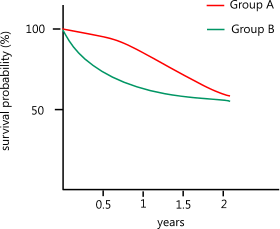• Comparing the entire curves provides more power
• In order to compare the entire curves, the Mantel-Haenszel test (also called the log-rank test) can be used
• To compare curves with the Mantel-Haenszel test, a 2 X 2 contingency table is created each time there is an event
• The formulas below are then used to derive the test statistic• If the two curves cross at any point, then the Mantel-Haenszel test is unlikely to be significant
• In this situation, other tests can be used which are more sensitive (ex. generalized Wilcoxon rank test)

• MANTEL-HAENSZEL (MH) TEST

• Overview
• The MH test is often used to compare stratified, categorical data. It can also be used to compare survival curves (see Kaplan-Meier estimator)
• To use the MH test, data is first stratified according to a particular criteria (ex. age: 20 - 30 years, 31 - 40 years, 41 - 50, etc.)
• A contingency table like the one below is then constructed for each strata. The order of the rows and columns does not matter.

•• For each contingency table, the number of observed and expected outcomes in cell a are calculated
• The number of observed outcomes is simply the summation of cell a for all the contingency tables (a+a+a...)
• The expected outcome for cell a is calculated by taking the proportion of patients in the first row and multiplying it by the proportion of patients in the first column. This product is then multiplied by the total number of patients. These values are then summed across all tables.

•• The MH test statistic and variance of the test statistic can then be calculated using the formulas below. The MH test statistic follows a chi-squared distribution with 1 degree of freedom

•• Continuity correction
• The 0.5 that is subtracted from the numerator in the Mantel-Haenszel test is called a "continuity correction." Some statisticians use it while others do not. It reduces the test statistic so that a significant results is harder to achieve. The reasoning behind the continuity correction is that a binomial outcome can only take on an integer value, but a chi-squared distribution approximates a continuous distribution. By subtracting 0.5 from the numerator, the numerator then assume the most conservative value for the integer in a continuous distribution.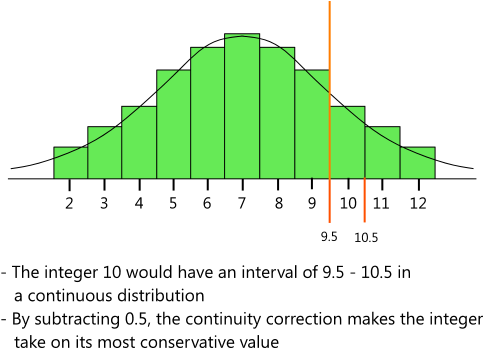• MEDIAN

• The median of a group of numbers is the number where half of the values are less than the median and half of the values are more than the median
• The median is useful because it is less sensitive to extreme values. Extreme values can distort the arithmetic mean
• Example:
• Group of values: 2, 4, 5, 6, 9, 22, 1000
• Median = 6
• Arithmetic mean = 149
• In most circumstances, the median will be a more meaningful measure of this group of numbers

• If the group of numbers has an even number of values, then the median is calculated by taking the two numbers in the middle and averaging them
• Example:
• Group of values: 2, 4, 5, 6, 9, 22, 30, 80
• Median = 6 + 9 / 2 = 15

• NORMAL DISTRIBUTION (GAUSSIAN DISTRIBUTION)

• The normal distribution is the most widely used continuous distribution in statistics
• It is also called the "Gaussian distribution" (after the mathematician who developed it, Karl Friedrich Gauss) and the "bell-shaped curve." When the mean is 0 and the standard deviation is 1, the normal distribution is called the "standard normal curve"
• The theory behind the normal distribution is that when a variable is sampled randomly from a population, the value of the variable will vary from individual to individual. Random factors will influence the variable with some pushing it higher, and some pushing it lower. If enough measurements are taken, the frequency distribution of the values will assume the shape of the normal curve.
• Distributions that are not normal can also be transformed so that they are approximately normal. For example, some factors affect a variable in a multiplicative manner. This can cause distributions that are skewed. By taking the logarithm of the values, the distribution will often be transformed into a normal distribution.
• The normal distribution also has the property that the area within one standard deviation of either side of the mean encompasses 68.3% of the values, and the area within two standard deviations encompasses 95% of the values

• The normal curve

•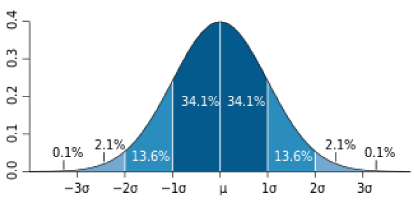•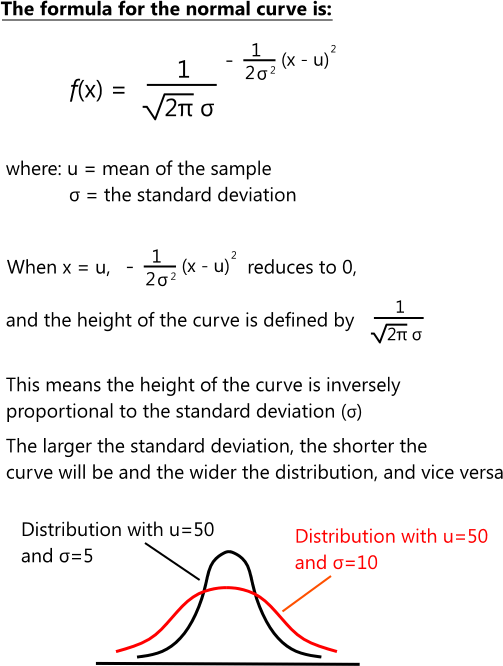• PARAMETRIC AND NONPARAMETRIC STATISTICS

• Parametric statistics
• Parametric statistics are methods used to analyze parametric data. Parametric data is of the interval or ratio class and its variance has an assumed distribution (e.g. normal, F-distribution). The name is derived from the fact that parametric data is sampled from a population that has an assumed set of "parameters."
• Examples of parametric data include temperature, weight, cholesterol levels, and blood pressure
• Examples of parametric tests include student's t-test and analysis of variance

• Nonparametric statistics
• Nonparametric statistics are methods used to analyze nonparametric data. Nonparametric data is of the ordinal type and its variance does not have an assumed distribution.
• Parametric data can be converted to nonparametric data and nonparametric statistical tests can be performed on the data. The opposite is not true.
• In some cases, it may be appropriate to use nonparametric methods on parametric data (e.g. small sample sizes, normal distribution cannot be assumed)
• In most cases, a positional metric like the median is preferred when working with nonparametric data. Nonparametric data intervals have no inherent meaning so metrics like the mean do not have consistent and meaningful value.
• Examples of nonparametric data include a pain scale that goes from 0 - 10, frequency counts, and Likert scales (strongly agree, agree, neutral, disagree, etc.)
• Examples of nonparametric tests include the Wilcoxon rank-sum test, Kruskal-Wallis test, and the sign test

• PERMUTATIONS AND COMBINATIONS

• Permutations
• Permutations and combinations are used in some statistical calculations (ex. Fisher's exact test)
• A permutation is the number of ways of selecting k items from a population of n items where the order of selection is important (i.e. ABC is considered different from BCA)• Combinations
• A combination is the number of ways of selecting k items from a population of n items where the order of selection is not important (i.e. ABC is considered the same as BCA)• PROBABILITIES

• Laws of probabilities

•• RECEIVER OPERATING CHARACTERISTIC (ROC) CURVES

• Overview
• In statistics, ROC curves are typically used to quantify the predictive value of a screening test or screening criteria. ROC curves are useful when the screening test has a continuous distribution or if a set of screening criteria are being tested.
• A ROC curve is a plot of the Sensitivity of a test (y-axis) vs 1 - Specificity of a test (x-axis). It can also be thought of as a plot of true positives (sensitivity) vs false positives (1 - specificity).
• The area under the ROC curve (also called the "C statistic") is defined as the probability that a person with the condition will have a higher score (or greater criteria) when compared to someone without the condition. A test with an area of 0.50 would have no meaning since it would equate to a 50% probability of having the condition.
• The greater the ROC curve area, the better the test is at predicting a condition. The table below gives generally accepted ranges for interpreting ROC curve areas. The ROC curve areas of two different tests can also be compared to determine if one test is significantly better than the other (see example below).

• Reference 
ROC curve area
(C statistic)
Test discrimination
< 0.60 Poor
0.60 - 0.75 Moderate
> 0.75 Good

• Example:
• Researchers are trying to determine if adding a coronary artery calcium (CAC) scores to a set of criteria for predicting heart disease (smoking, hypertension, high cholesterol, diabetes, family history) increases the predictive value of the criteria
• They recruit 1000 subjects and measure their values for each criteria. They then perform a heart catheterizations on every subject to determine if they have heart disease.
• Patients are grouped by the number of criteria (1 - 6) that they are positive for (a CAC score > 400 is considered positive for the CAC criteria)
• The sensitivity and specificity for the presence of heart disease in each group is then calculated

Criteria positive Sensitivity Specificity
1 100% 0
2 90% 54%
3 86% 65%
4 76% 75%
5 40% 82%
6 0% 100%

• The ROC curve below is drawn using the data above
• The data for this ROC curve used all six criteria (smoking, hypertension, high cholesterol, diabetes, family history, CAC score)• The area under this ROC curve is 0.77
• Another ROC curve is constructed from data that did not include the CAC score. The area under this ROC curve is 0.69.
• Researchers compare the two ROC curves and find that there is a significant difference in their areas
• They come to the conclusion that adding CAC scores to their heart disease risk model significantly improves its predictive value

• STANDARD DEVIATION

• Overview
• The standard deviation is a measure of spread. A group of data that have a wide range of values will have a larger standard deviation when compared to a group of data that has a narrow range of values.
• Steps for calculating the standard deviation:
• 1. Sum the squares of the difference for each data point from the mean of the data points
• 2. Divide the sum from step 1 by the total number of data points minus one (n-1)
• 3. Take the square root of this value
• Standard deviation formula:

•• Properties of the standard deviation
• If a set of values is sampled from a population that is assumed to have a normal distribution, then the standard deviation has several useful properties
• 68% of the values from that population will fall within -1 to +1 standard deviations of the mean
• 95% of the values from that population will fall within -2 to +2 standard deviations of the mean
• 99.7% of the values from that population will fall within -3 to +3 standard deviations of the mean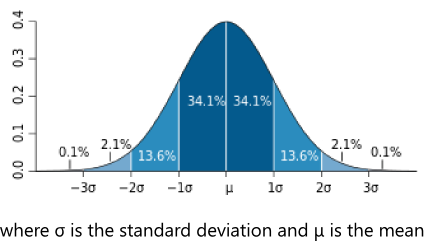• The standard deviation and its square (s² = variance) are used in a number of inferential statistical calculations

• STANDARD ERROR OF THE MEAN (SEM)

• The standard error of the mean (also referred to as the standard error) is a measure that is frequently used in inferential statistics
• The SEM can be interpreted as follows:
• 1. Take a random sample of size n from a population and calculate the mean
• 2. Repeat this process over and over by taking random samples of size n from the same population
• 3. Average the sample means
• 3. The average of the sample means will have a standard deviation equal to the SEM

• The SEM is calculated as follows:

•• BIBLIOGRAPHY

• 1 - Fundamentals of Biostatistics, 7th ed. ISBN-13: 978-0538733496
• 2 - Biostatistics: The Bare Essentials, 3rd ed. ISBN-13: 978-1550093476
• 3 - Intuitive Biostatistics, 2nd ed. ISBN-13: 978-0199730063
• 4 - Online Statistics Education: A Multimedia Course of Study (http://onlinestatbook.com/). Project Leader: David M. Lane, Rice University
• 5 - PMID 29049590 - Discrimination and Calibration of Clinical Prediction Models: Users' Guides to the Medical Literature, JAMA (2017)